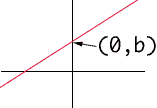Date: Thu, 15 Apr 1999 14:17:39 -0600 (CST)
Subject: slopes

Name: Selena
Who is asking: Student
Level: Middle

Question:
When solving a problem on slopes,and in the formula y=mx+b what do the M and B stand for? Why M and B?

Hi Selena
 In the standard equation for a line, y = mx + b, m designates the slope of the line, and b the y-intercept, that is b is the second coordinate of the point where the line crosses the y-axis. Why m and b is an interesting question. We have been asked that question a number of times and the short answer is that we don't know. Look here for a more detailed answer.Cheers
Penny

Go to Math Central

To return to the previous page use your browser's back button.